# `20191024a`

Testing the D2a and D2b bins for dynamic DAC for TGC.

Weird glitches at regular intervals on D2a and C2w bins.

# Images of the Experiment

## Others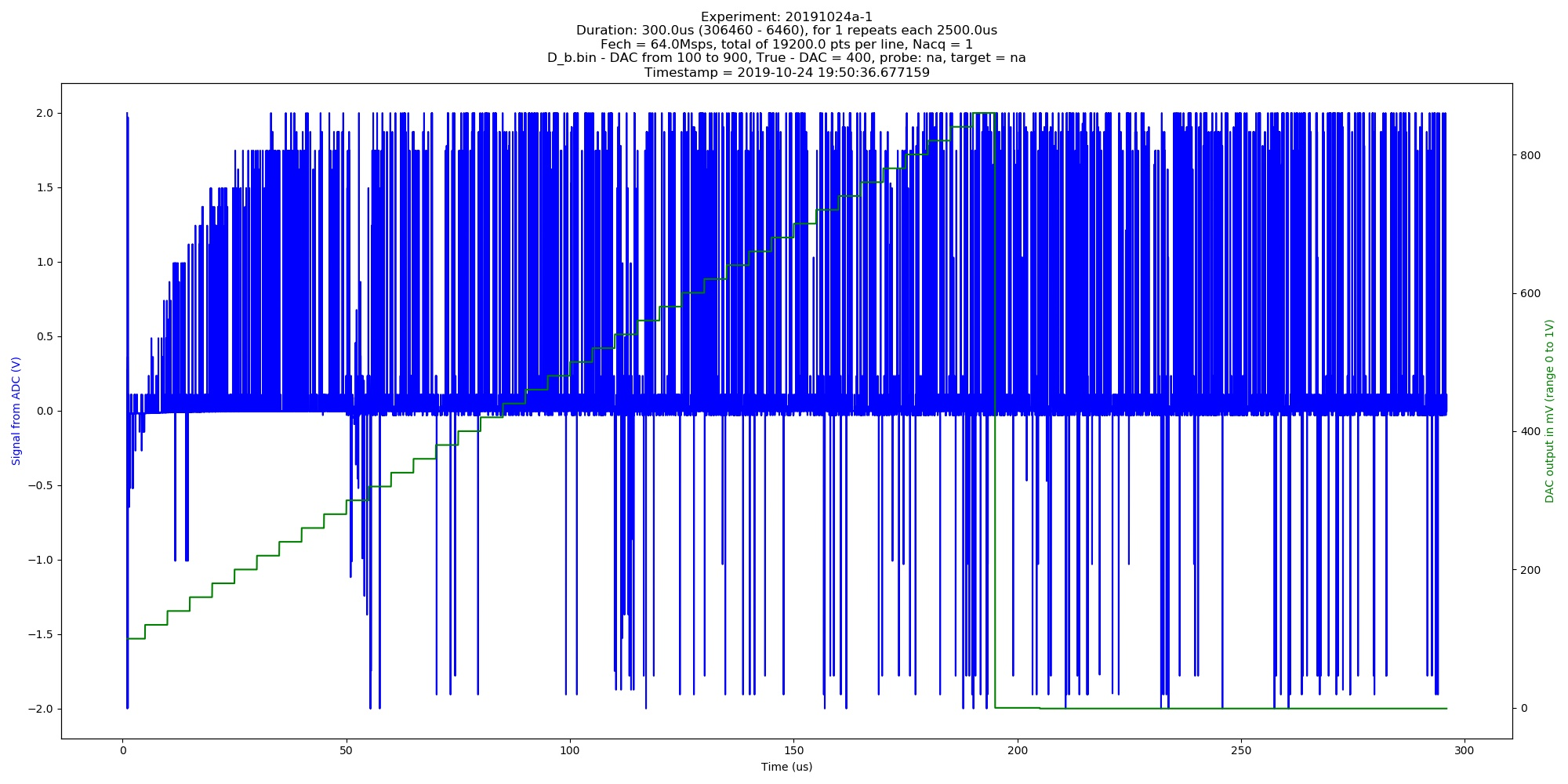Automated image of 20191024a experiment. Db.bin - DAC from 100 to 900, True - DAC = 400 (category: _graph).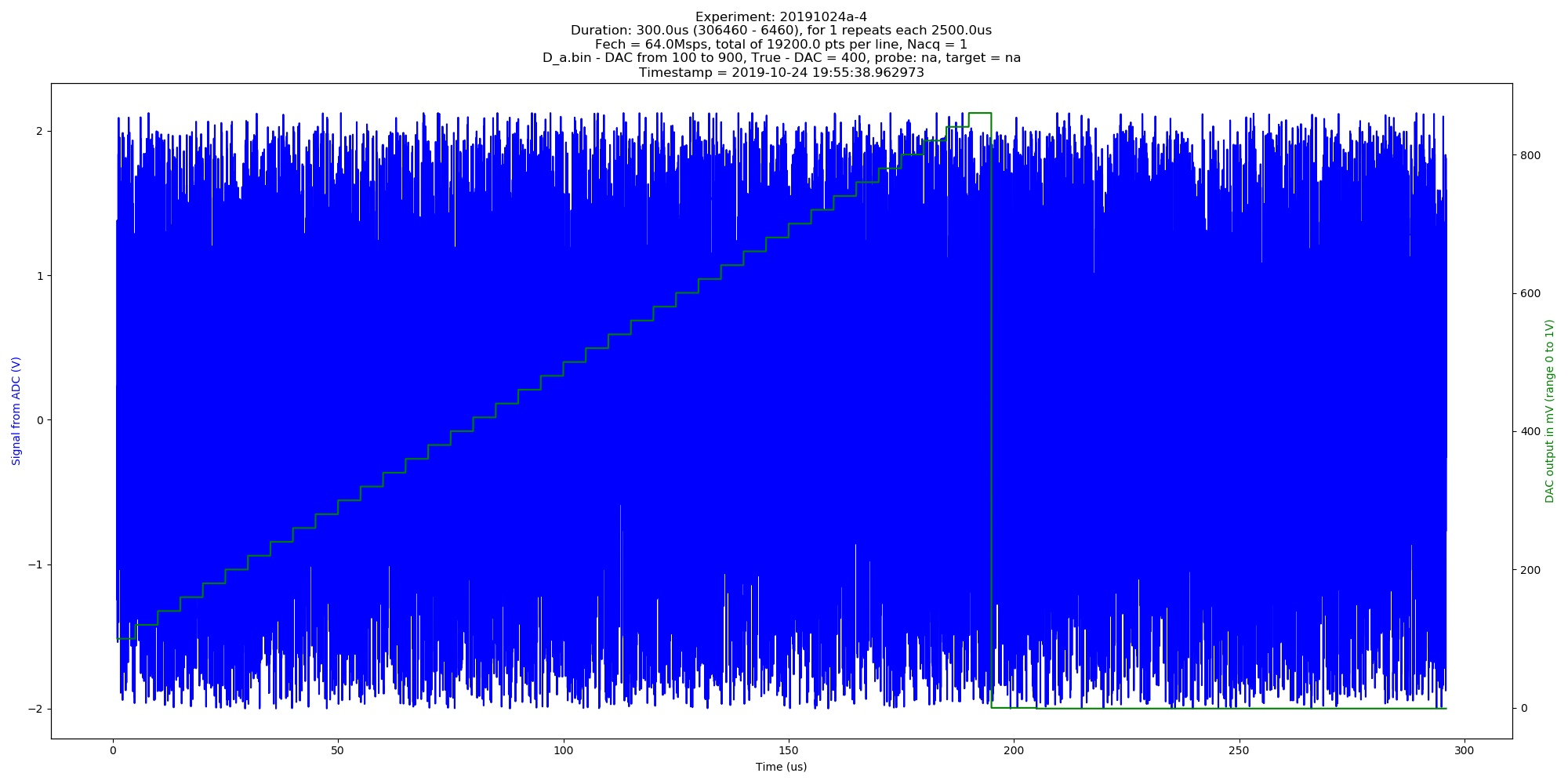Automated image of 20191024a experiment. Da.bin - DAC from 100 to 900, True - DAC = 400 (category: _graph).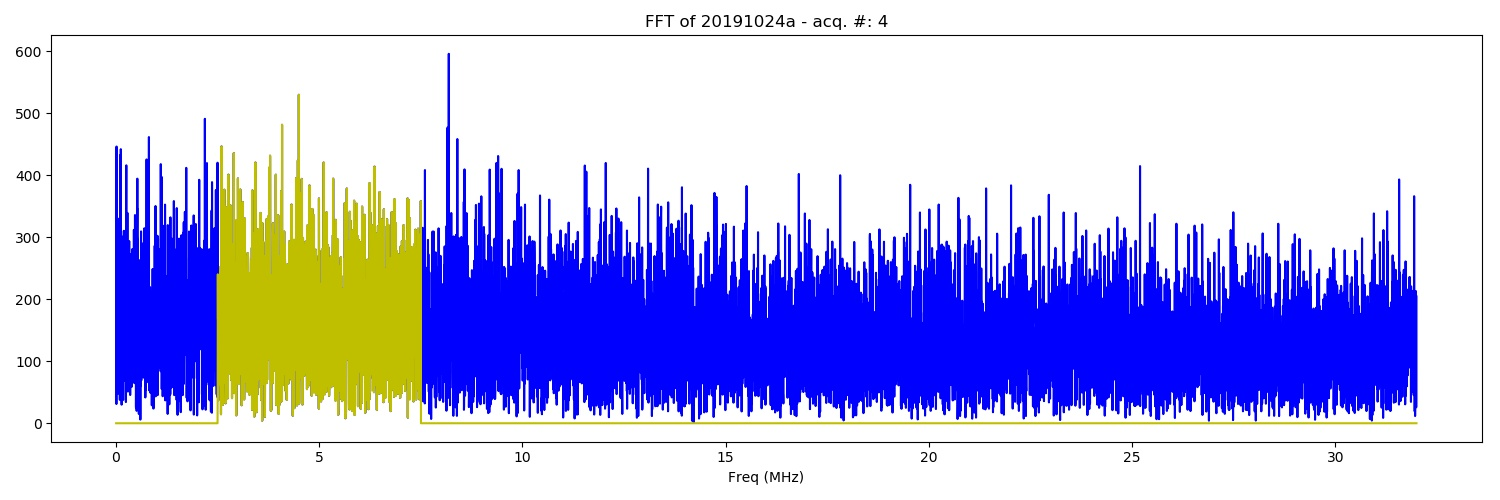FFT of the of 20191024a experiment. Da.bin - DAC from 100 to 900, True - DAC = 400 (category: _FFT).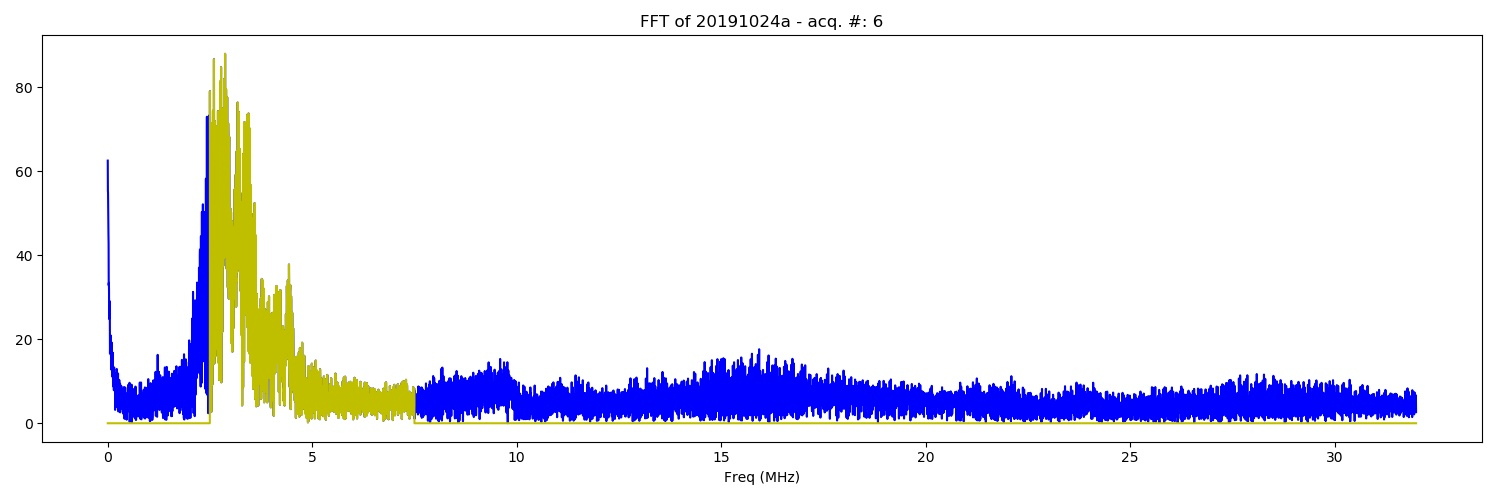FFT of the of 20191024a experiment. C2w.bin - DAC from 100 to 900, True - DAC = 400 (category: _FFT).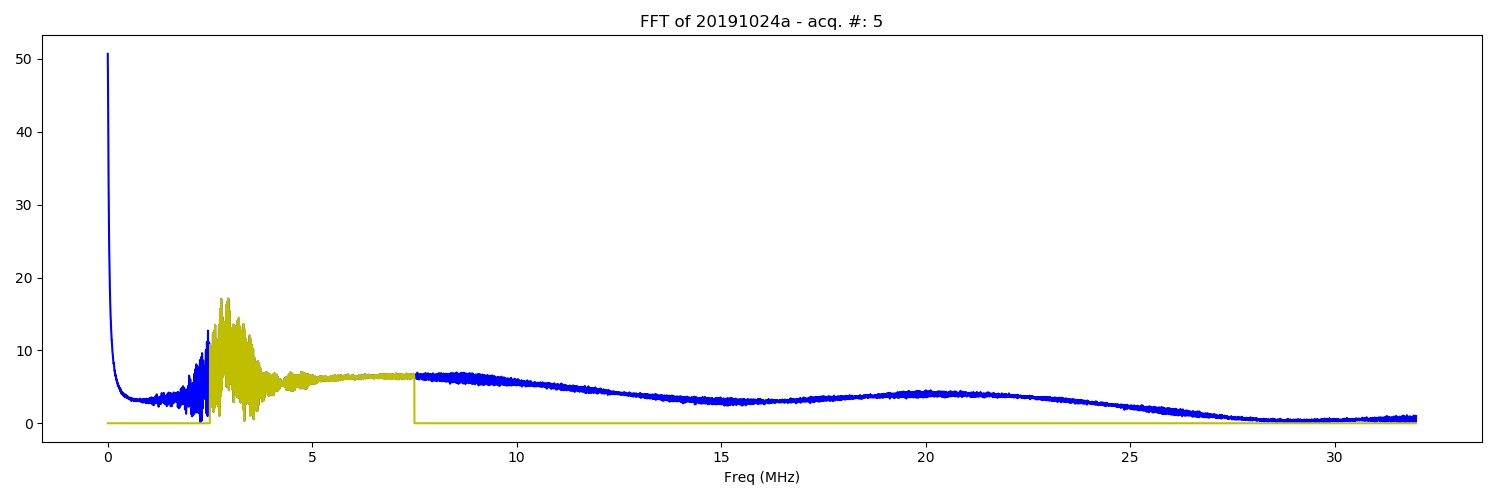FFT of the of 20191024a experiment. Da.bin - DAC from 100 to 900, True - DAC = 400 (category: _FFT).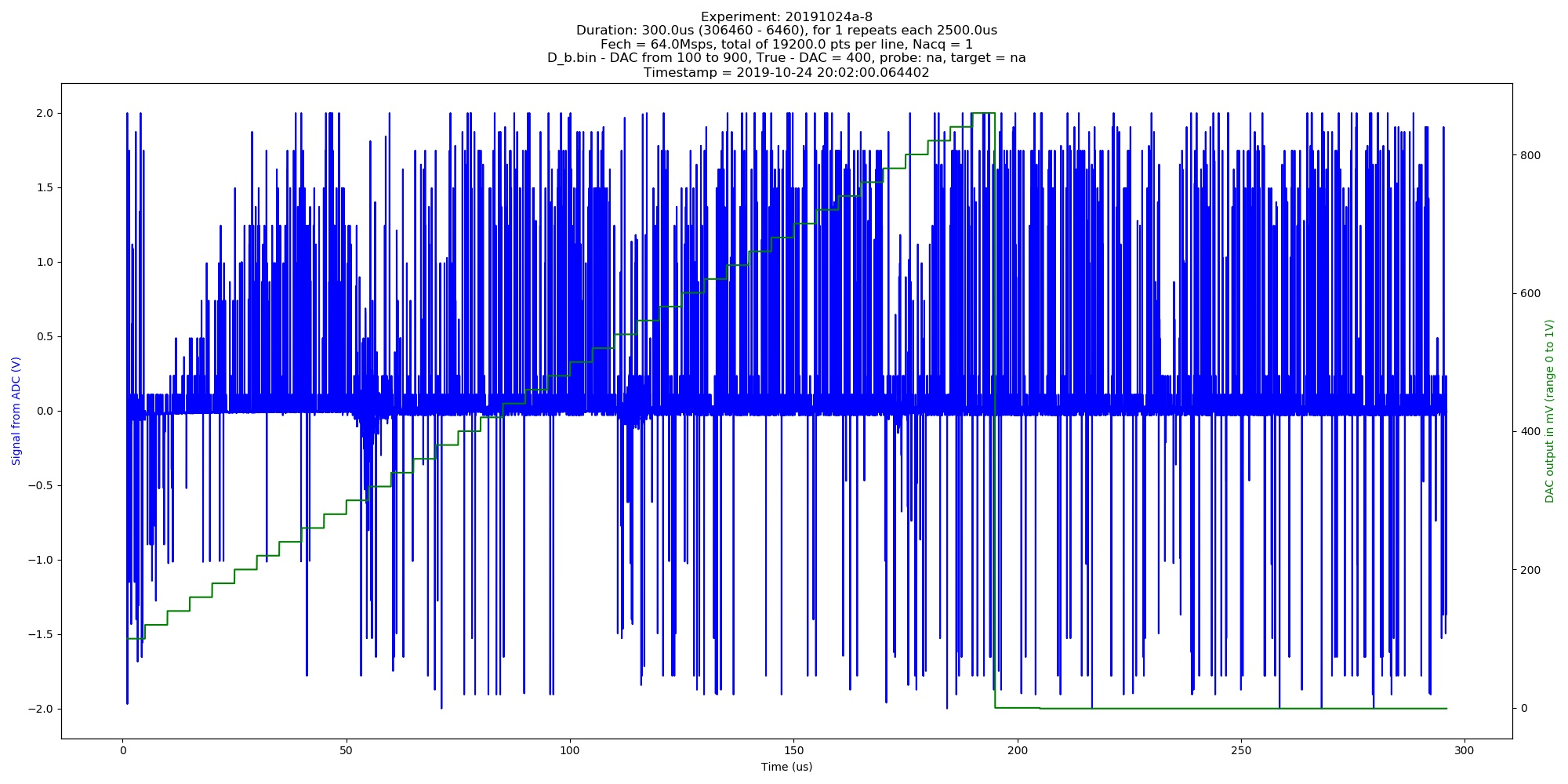Automated image of 20191024a experiment. Db.bin - DAC from 100 to 900, True - DAC = 400 (category: _graph).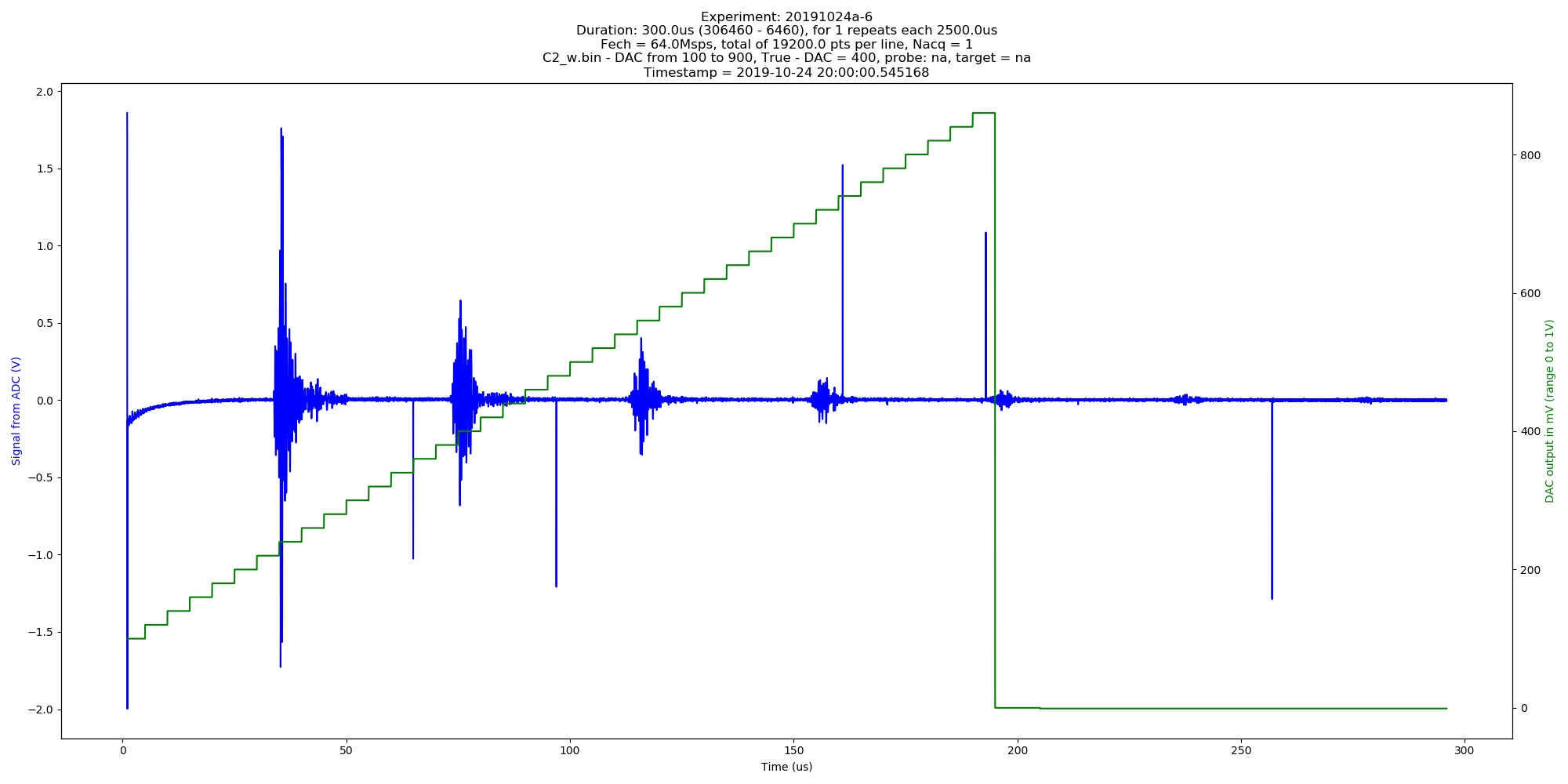Automated image of 20191024a experiment. C2w.bin - DAC from 100 to 900, True - DAC = 400 (category: _graph).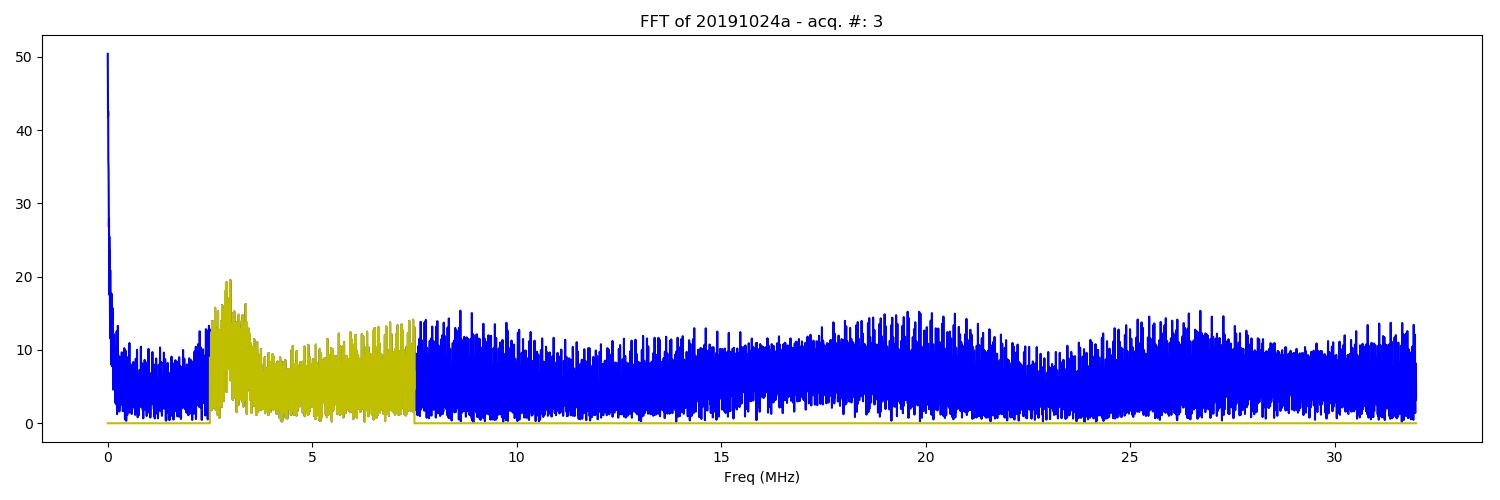FFT of the of 20191024a experiment. C2w.bin - DAC from 100 to 900, True - DAC = 400 (category: _FFT).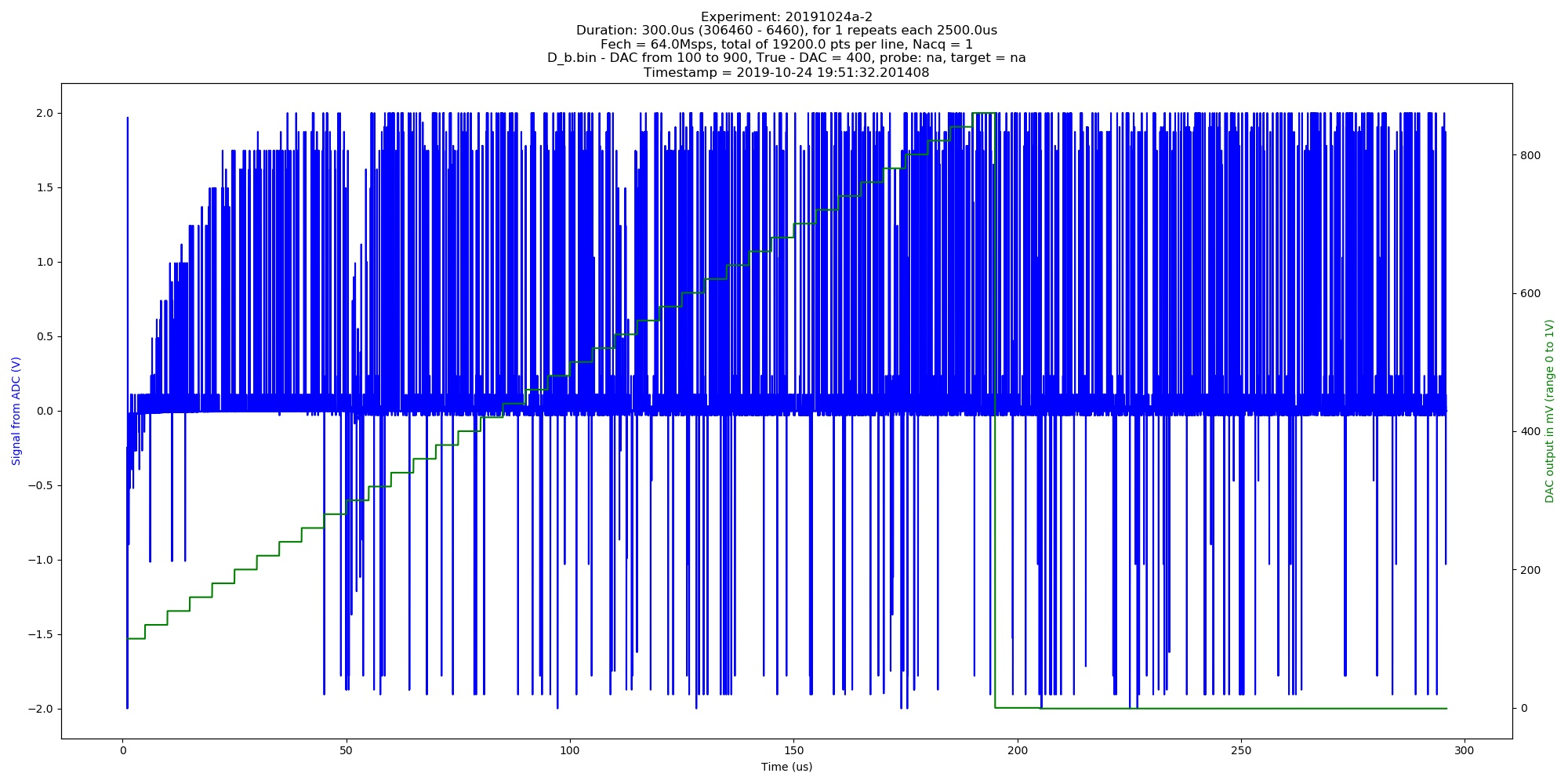Automated image of 20191024a experiment. Db.bin - DAC from 100 to 900, True - DAC = 400 (category: _graph).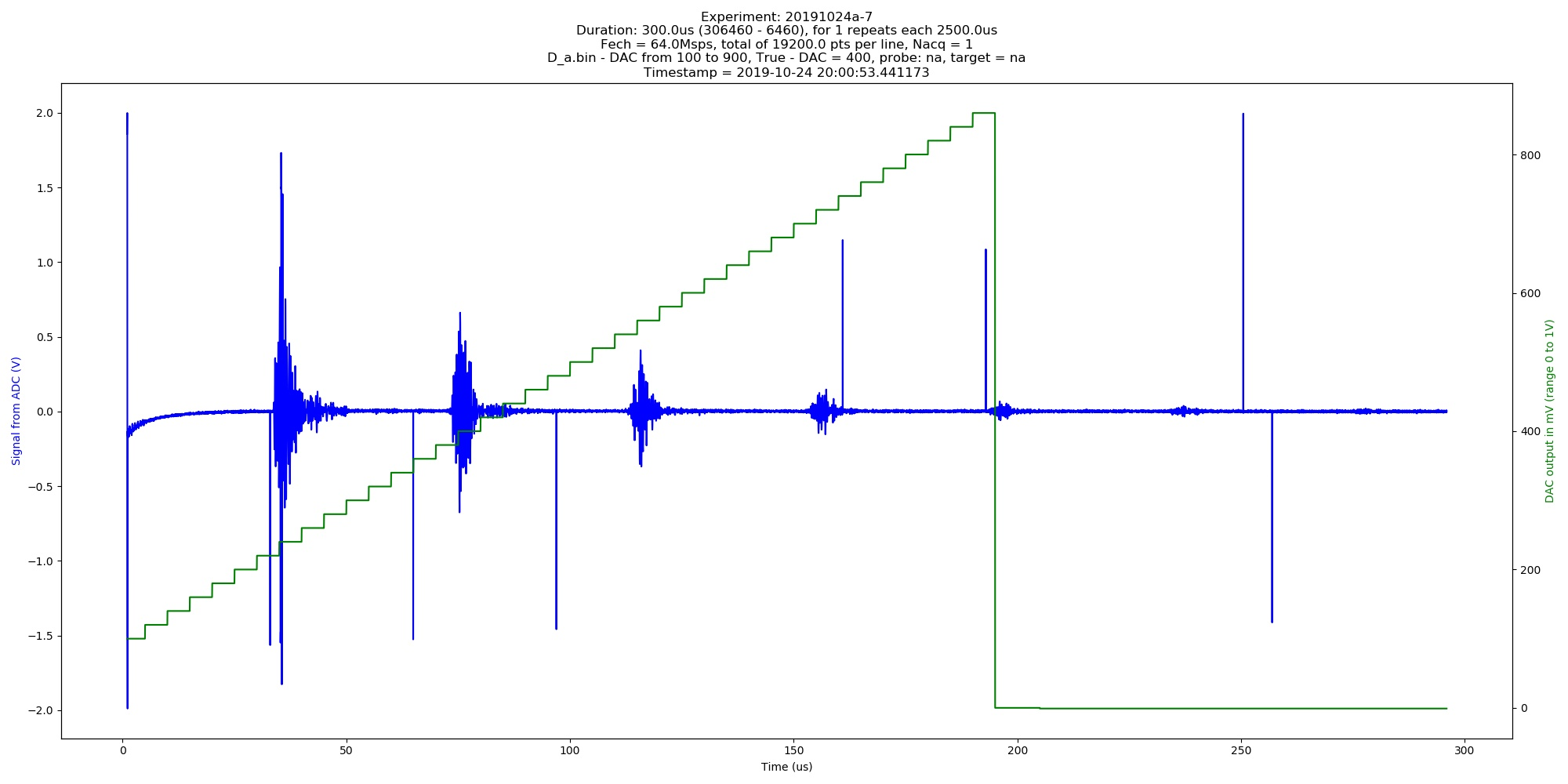Automated image of 20191024a experiment. Da.bin - DAC from 100 to 900, True - DAC = 400 (category: _graph).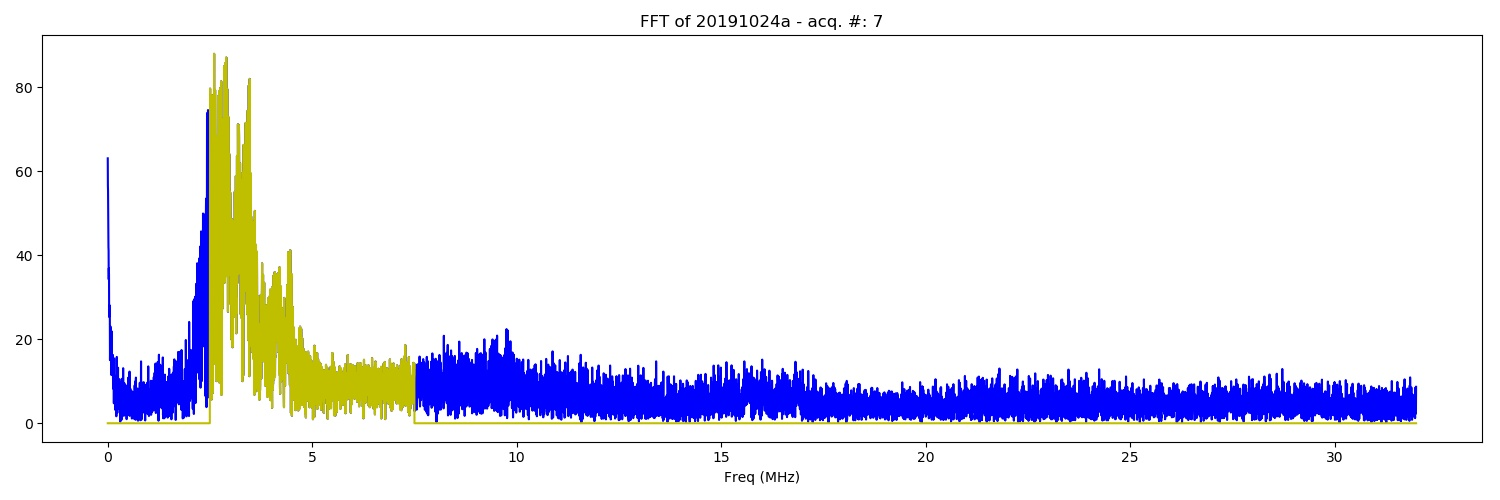FFT of the of 20191024a experiment. Da.bin - DAC from 100 to 900, True - DAC = 400 (category: _FFT).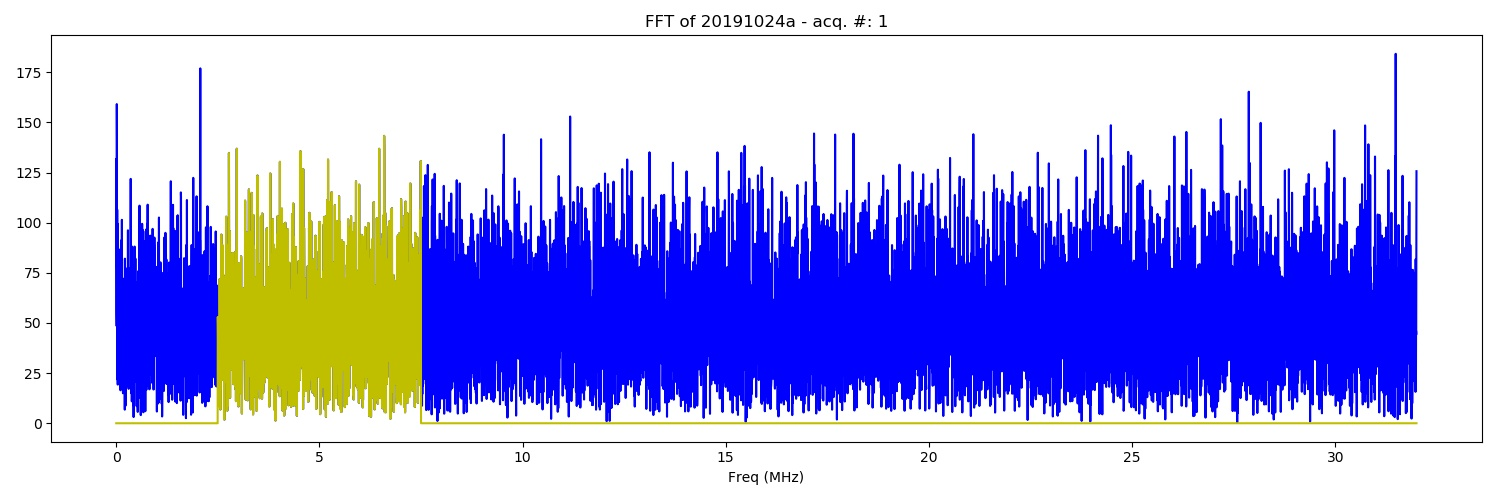FFT of the of 20191024a experiment. Db.bin - DAC from 100 to 900, True - DAC = 400 (category: _FFT).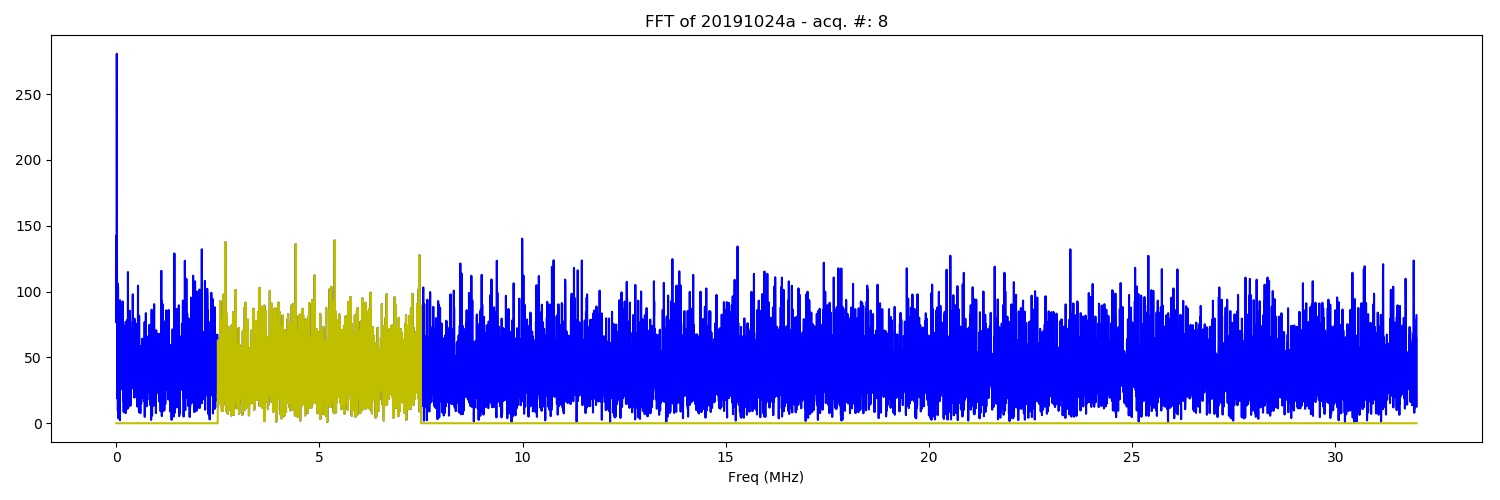FFT of the of 20191024a experiment. Db.bin - DAC from 100 to 900, True - DAC = 400 (category: _FFT).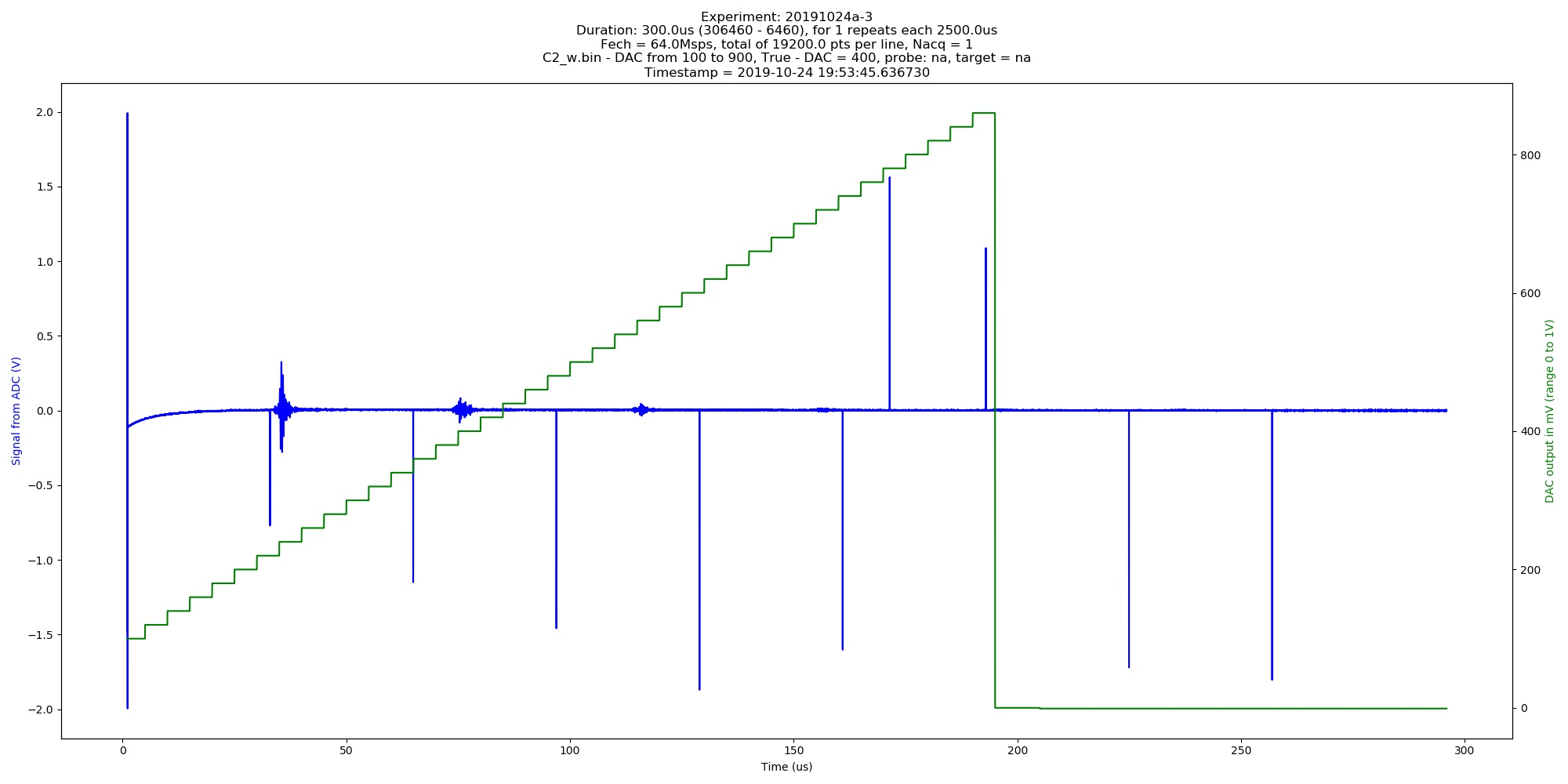Automated image of 20191024a experiment. C2w.bin - DAC from 100 to 900, True - DAC = 400 (category: _graph).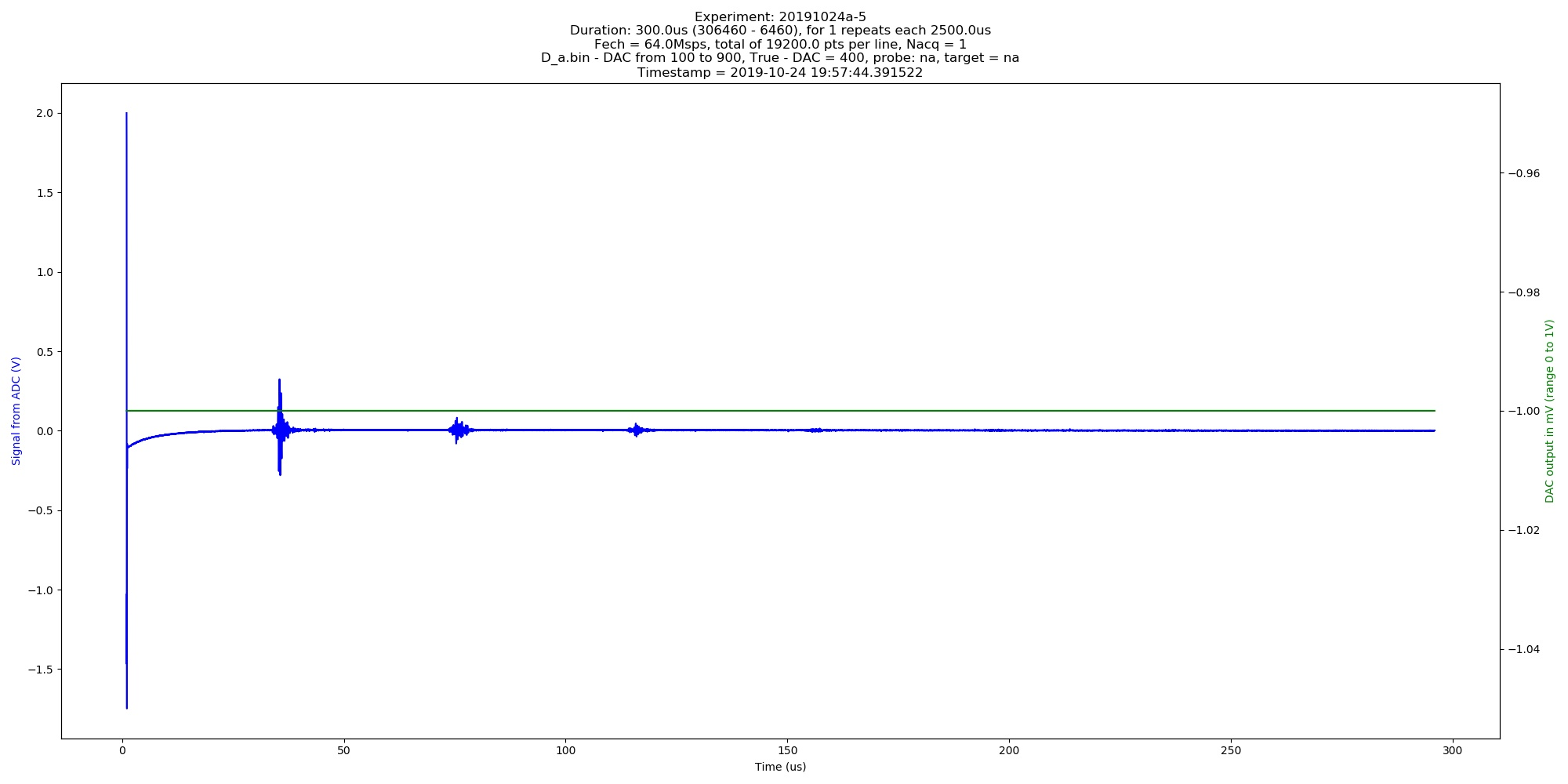Automated image of 20191024a experiment. Da.bin - DAC from 100 to 900, True - DAC = 400 (category: _graph).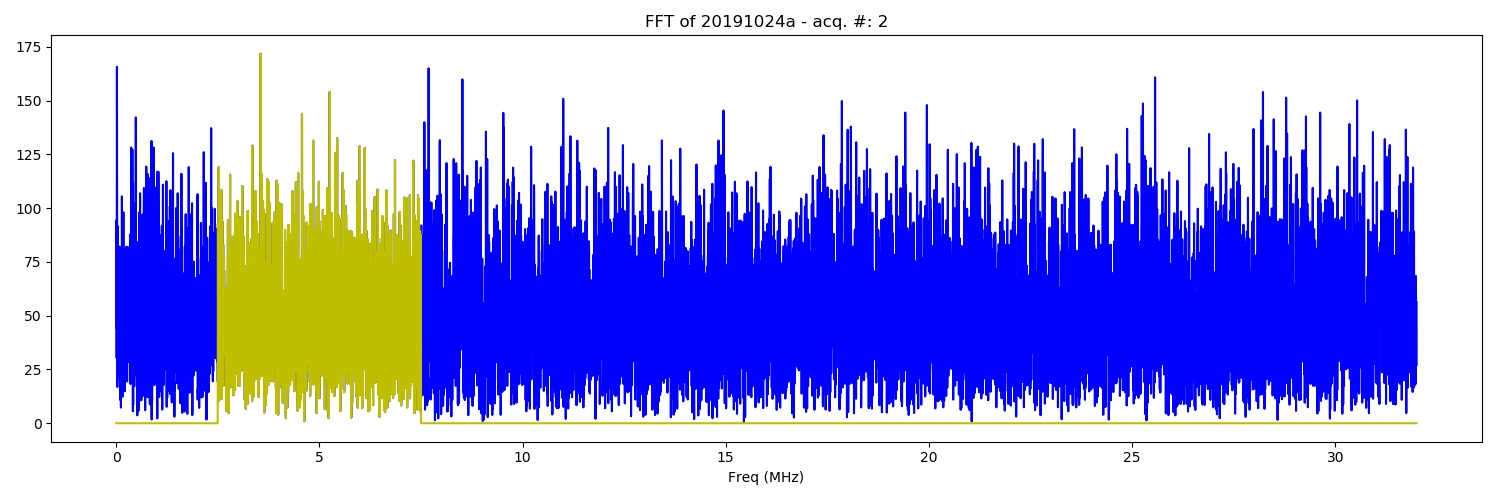FFT of the of 20191024a experiment. Db.bin - DAC from 100 to 900, True - DAC = 400 (category: _FFT).